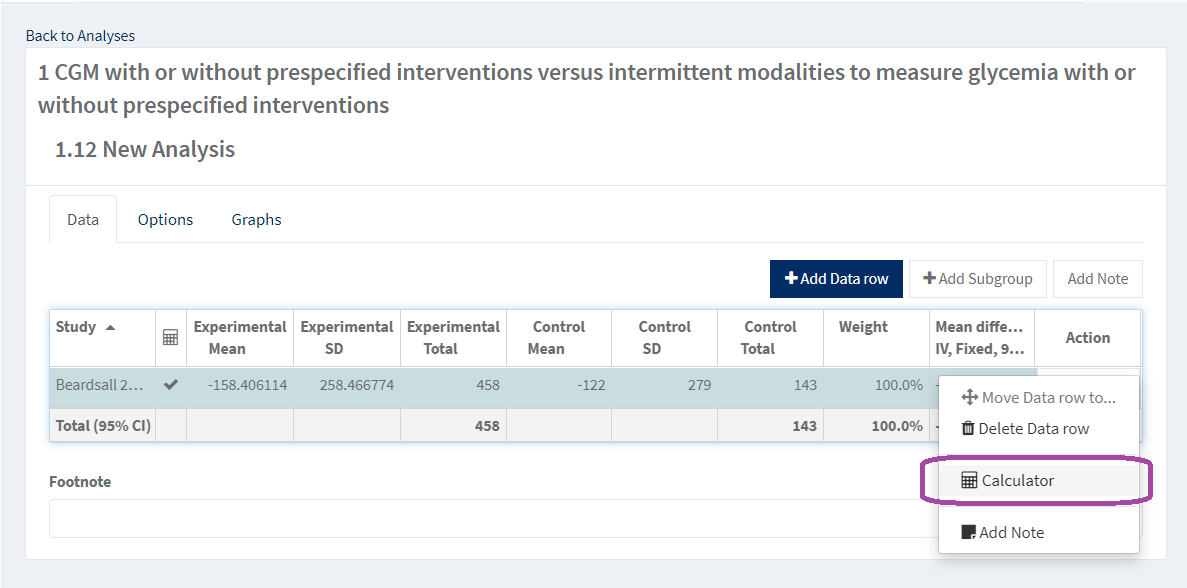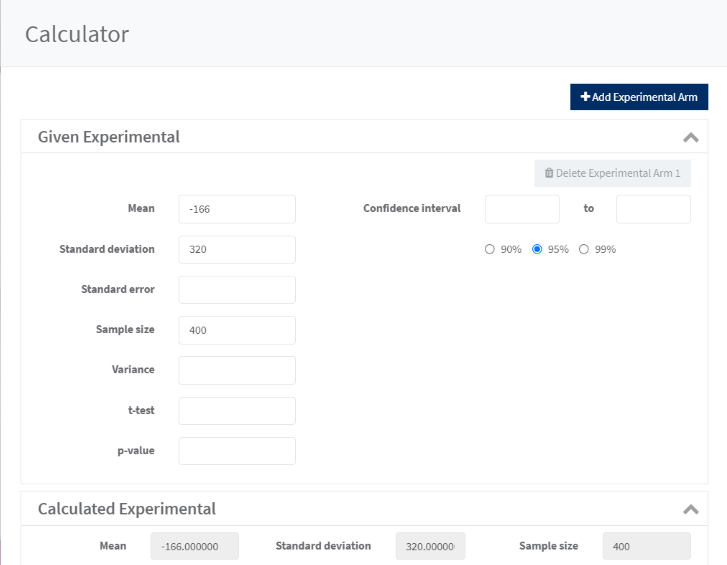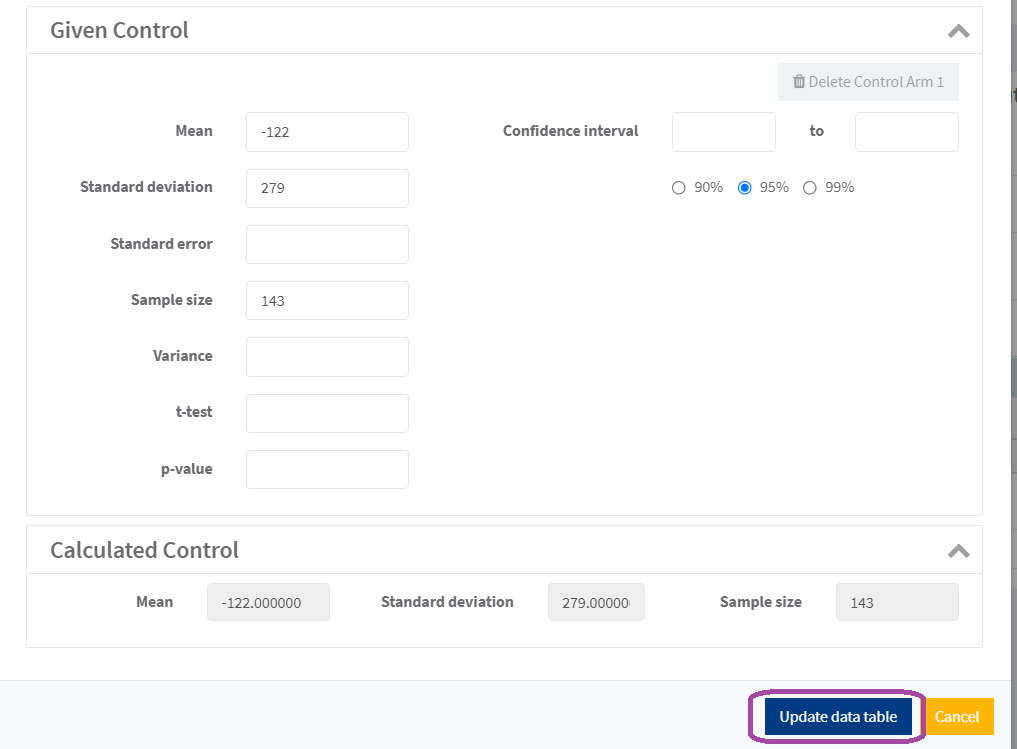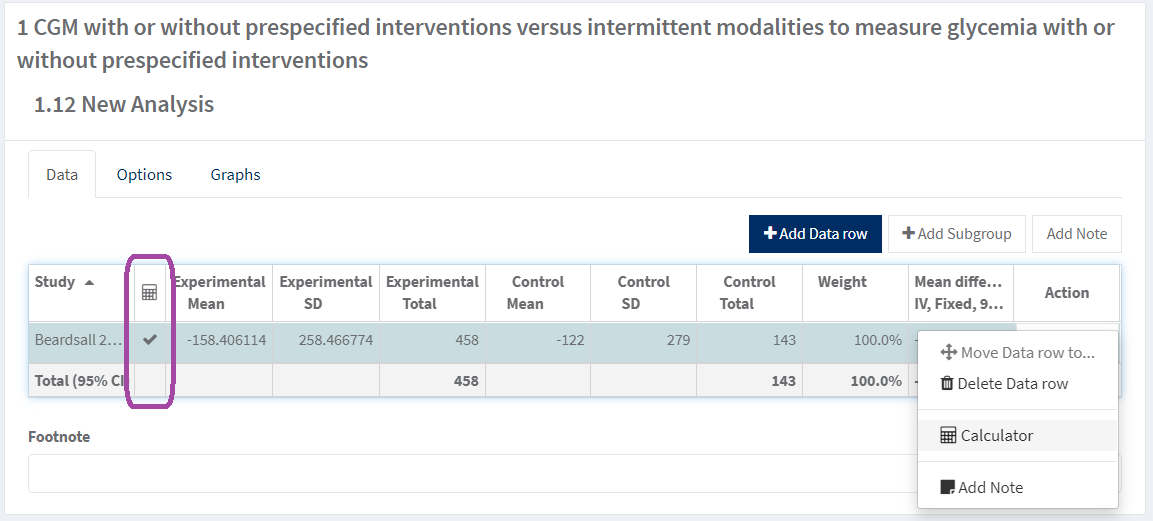# Calculator

The calculator function in RevMan Web can be used to transform raw data extracted from study reports when it does not match the format required for meta-analysis.

There are various scenarios where this might be needed, and the approach in RevMan differs depending on the type of data available and the desired output. Common uses include:

• transforming standard errors or confidence intervals for group means into standard deviations (within a continuous analysis)
• combining continuous data for two related arms into a single data row (within a continuous analysis)
• entering two-by-two data in the same analysis as pre-calculated odds ratios or risk ratios (using a dichotomous GIV analysis)
• entering experimental and control group data in the same analysis as pre-calculated mean differences (using a GIV continuous analysis)

RevMan Web saves the data entered via the calculator and the transformed data output which makes the process more transparent, and the data easier to check and manipulate.

## Access calculator

In the analysis result data table, you can access the calculator for a specific result by selecting the Action drop down menu and choosing Calculator. Note that there is no calculator available for analyses with dichotomous data type.## Enter data

In the given panel you can enter the data reported in the study. Note that some of the fields have validation to ensure you are inputting the correct data. If you enter inconsistent data RevMan will use the most relevant value and ignore the other values. There is validation in place to help you detect if you are entering data on the wrong format.## Update data table

When you have enough given values the calculated values will be shown, and you can choose to update the data table which will populate the result data table with the calculated values.## Access previously calculated data

Analysis results calculated using the calculator will be saved when you click ‘Update data table’ and this will be indicated in the result data table next to the study ID (see figure below). All the inputted data in the calculator will be saved, not only data used for the calculations.Note that data that is saved by the calculator will be deleted if:

• the corresponding result row is updated or overwritten in RevMan Web or RevMan 5
• the output effect measure is changed in RevMan Web or RevMan 5 (e.g. from mean difference to standardised mean difference), or if the data type is changed in RevMan 5
• the study ID for the result row is changed in RevMan Webthe analysis containing saved data is moved to another analysis group (comparison) in RM5
• the analysis subgroup containing the saved data is dismantled in RM5
• A warning will appear only in RMW and not in RM5 when one of the above actions is taken in RevMan Web that would result in saved data being deleted

## Combine arms

In some trials, more than one arm per group is reported. Then it will be useful to use the calculator to calculate a pooled mean and standard deviation. You do this by clicking ‘Add Experimental Arm’ or ‘Add Control Arm’. The calculator will then automatically pool the means and standard deviations when you have entered data for the arms. There is no limit to how many arms you can add. You can combine arms for analyses with continuous data type and for GIV analyses with contiuous data.